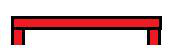* Log In to use the Calculate function * Become a Member!

###### Design of Steel Beams:Steel Beam Design

Angle Flexure Compactness
• Check the compactness of an angle shape in flexure.

Box Flexure Compactness
• Check the compactness of a box shape in flexure.
Rolled I-shape or Channel Flexure Compactness
• Check the compactness of a rolled I-shape or channel in flexure and calculate radius of gyration of the compression area.
Pipe or Round HSS Flexure Compactness
• Check the compactness of a pipe or round HSS in flexure.
Lp, Lr of Compact I-shapes and Channels
• Calculate the unbraced length limit state for yielding, Lp and for inelastic lateral-torsional buckling, Lr, for compact I-shapes and channels.
Lp, Lr of Noncompact and Slender I-shapes and Channels
• Calculate the unbraced length limit state for yielding, Lp and for inelastic lateral-torsional buckling, Lr, for noncompact and slender I-shapes and channels.
Lateral-torsional buckling modification factor, Cb
• Calculate the lateral-torsional buckling modification factor for nonuniform moment diagrams when both ends of the segment are braced.
•
Flexural Strength - Compact I-shapes and Channels
• Calculate the flexural strength (maximum moment) of a compact I-shape or channel.
Flexural Strength - CW and NCF or SF I-shapes and Channels
• Calculate the flexural strength (maximum moment) of an I-shape or channel with a compact web and noncompact or slender flanges.
Flexural Strength - NCW and SW I-shapes and Channels
• Calculate the flexural strength (maximum moment) of an I-shape or channel with a noncompact  or slender web.
Flexural Strength - Minor Axis of I-shapes and Channels
• Calculate the flexural strength (maximum moment) of an I-shape or channel bent around the minor axis.
Flexural Strength - Rectangular HSS and Box Shapes
• Calculate the flexural strength (maximum moment) of a box shape or rectangular HSS.
Flexural Strength - Tees
• Calculate the flexural strength of a tee loaded in the plane of symmetry.
Flexural Strength - Double Angles
• Calculate the flexural strength of a double angle loaded in the plane of symmetry.
Flexural Strength - Angles
• Calculate the flexural strength (maximum moment) of an angle.
Flexural Strength - Pipe or Round HSS
• Calculate the flexural strength (maximum moment) of a pipe or round HSS.
Shear - Angles
• Calculate the shear strength of an angle.
Shear - Rolled I-shapes and Channels
• Calculate the shear strength of a rolled I-shape or channel.
Shear - Other Than Rolled I-shapes, Channels and HSS
• Calculate the shear strength of other than rolled I-shapes, channels and HSS.
Shear - Rectangular HSS and Box
• Calculate the shear strength of a rectangular HSS or box shape.
Shear - Pipe and Round HSS
• Calculate the shear strength of a pipe or round HSS.
Shear - Minor Axis of I-shapes and Channels
• Calculate the shear strength of an I-shape or channel bent around the minor axis.
Flexural Strength - Rectangular or Round Bars
• Calculate the flexural strength of rectangular bars bent about either geometric axis and rounds.
Serviceability - Deflection
• Calculate the deflection for common load conditions of simple-span beams and I-shaped members and channels using load constants.
Shears and Moments
Beam Deflection
50 ksi I Shapes Lp and Lr Values
• US Standard Units Lp and Lr value tables for 50 ksi steel I shapes, including values of Rts, c, Lp, Lr, ASD and LRFD maximum moments at Lp and Lr in US standard Units for common 50 ksi doubly symmetric rolled I-shapes.
345 MPa I Shapes Lp and Lr Values
• Metric Units Lp and Lr value tables for 345 MPa steel I shapes, including values of Rts, c, Lp, Lr, ASD and LRFD maximum moments at Lp and Lr in Metric Units for common 345 MPa doubly symmetric rolled I-shapes.
36 ksi I Shapes/Channels Lp and Lr Values
• US Standard Units Lp and Lr value tables for 36 ksi steel I shapes and channels, including values of Rts, c, Lp, Lr, ASD and LRFD maximum moments at Lp and Lr in US standard Units for common 36 ksi doubly symmetric rolled I-shapes.
248 MPa I Shapes/Channels Lp and Lr Values
• Metric Units Lp and Lr value tables for 248 MPa steel I shapes and channels, including values of Rts, c, Lp, Lr, ASD and LRFD maximum moments at Lp and Lr in Metric Units for common 248 MPa doubly symmetric rolled I-shapes.
Design Problem 1
• Find the lightest weight W section for a simple beam to carry the factored loading.

 Start Using The Calculations >>> Become A Member Now!